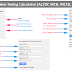Here, using this Circuit Breaker Rating Calculator, you can calculate the rating or capacity of different types of circuit breakers such as MCB, MCCB, ACB, and More. When you provide details of your electrical system the calculator will automatically recommend you the proper circuit breaker type, name, and their current and voltage ratings. It is designed to help users determine the appropriate rating for circuit breakers based on the power system they are working with.

Circuit Breaker Rating Calculator

## Circuit Breaker Rating Calculator

### How to Use This Calculator?

This calculator allows you to calculate circuit breaker ratings for DC Power Systems, AC single-phase, and AC three-phase power systems. So follow the below procedures to use this calculator,

For DC Power System:

1. Enter the "System Voltage" in volts (V) or kilovolts (KV) in the input field labeled "System Voltage." This value represents the voltage of your DC power system.

2. Enter the "Total Power to be Used" in watts (W) or kilowatts (KW) in the input field labeled "Total Power to be Used." This value represents the total power rating of the loads connected to your DC system.

3. Enter the "Safety Factor" as a percentage (%). The calculator recommends a safety factor of more than 25%. Enter this value in the input field labeled "Safety Factor."

4. Click the "Calculate" button to get the "Circuit Breaker Ratings." The result will be displayed below the form.

For Single Phase AC Power System:

1. Enter the "System Voltage" in volts (V) or kilovolts (KV) in the input field labeled "System Voltage."

2. Enter the "Total Power to be Used" in watts (W) or kilowatts (KW) in the input field labeled "Total Power to be Used." This value represents the total power rating of the loads connected to your single-phase AC system.

3. Enter the "Power Factor (pf)" as a decimal between 0 and 1. This value should be less than 1 and indicates the power factor of your system. Enter this value in the input field labeled "Power Factor (pf)."

4. Enter the "Safety Factor" as a percentage (%). The calculator recommends a safety factor of more than 25%. Enter this value in the input field labeled "Safety Factor."

5. Now, Click the "Calculate" button to get the "Circuit Breaker Ratings." The result will be displayed below the form.

For Three-Phase AC Power System:

1. Select the "Voltage Type" by choosing either "Line to Line Voltage (VLL)" or "Line to Neutral Voltage (VLN)" from the drop-down menu labeled "Select Voltage Type."

2. Enter the "System Voltage" in volts (V) or kilovolts (KV) in the input field labeled "System Voltage."

3. Enter the "Total Power to be Used" in watts (W) or kilowatts (KW) in the input field labeled "Total Power to be Used." This value represents the total power rating of the loads connected to your three-phase AC system.

4. Enter the "Power Factor (pf)" as a decimal between 0 and 1. This value should be less than 1 and indicates the power factor of your system. Enter this value in the input field labeled "Power Factor (pf)."

5. Enter the "Safety Factor" as a percentage (%). The calculator recommends a safety factor of more than 25%. Enter this value in the input field labeled "Safety Factor."

6. Click the "Calculate" button to get the "Circuit Breaker Ratings." The result will be displayed below the form.

### What is Safety Factor?

The safety factor is also known as the safety margin. It is an additional percentage added to the calculated circuit breaker rating to ensure that the circuit breaker can handle the load safely and reliably.

For example, if the calculated circuit breaker rating based on the load requirements is 100 Amperes, applying a 25% safety factor would increase it to 125 Amperes. This ensures that the circuit breaker is not operating near its maximum capacity and can handle unexpected surges or fluctuations in the electrical system without tripping unnecessarily.

The specific value of the safety factor can vary depending on the application and the level of redundancy or reliability required. However, a common recommendation is to use a safety factor of more than 25%. This means that the calculated circuit breaker rating should be increased by at least 25% to provide the final recommended rating.

It is important to note that while the safety factor provides an additional margin of protection, selecting a circuit breaker with too high of a rating can lead to inefficiencies and higher costs. Therefore, it's essential to keep a balance between ensuring safety and avoiding excessive overrating. It is recommended to take advice from qualified electrical engineers or professionals to determine the appropriate safety factor for a particular circuit breaker application.

### Formula

Here are the formulas the above calculator uses to determine the current rating of the circuit breaker,

For the DC Power System

Circuit Breaker Current (Icb) = (Power / Voltage) * (1 + Safety Factor value / 100)

For the AC Single-Phase Power System

Circuit Breaker Current (Icb) = (Power / Voltage * Power Factor) * (1 + Safety Factor value / 100)

For the AC Three-Phase Power System(Line to Line Voltage)

Circuit Breaker Current (Icb) = (Power / 1.73 *Voltage * Power Factor) * (1 + Safety Factor value / 100)

For the AC Three-Phase Power System(Line to Neutral Voltage)

Circuit Breaker Current (Icb) = (Power / 3 *Voltage * Power Factor) * (1 + Safety Factor value / 100)

Thank you for visiting the Website. Keep visiting for more Updates.

Circuit Breaker Rating Calculator (AC/DC MCB, MCCB, and More)Reviewed by Author on August 04, 2023 Rating: 5